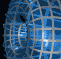Português | English Beyond 3D > Iced Cubes > Slicing 4D hypercube

# Slicing a four-dimensional hypercube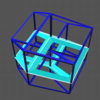This movie shows the orthographic view of a hypercube looking directly at a corner of the hypercube (i.e., along its long diagonal, see the movies of projections of cubes for more about the different views of the hypercube). In this movie, the hypercube is being sliced parallel to one of its cubical faces. The initial slice is one complete cube, and then this slice seems to translate across the projection to the opposite cube. During the intermediate stages, each face of the slice comes from one of the six cubes between the "top" and "bottom" cubes in the hypercube. During the slicing sequence, these faces each sweep out one of these six cubes.

` `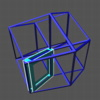This movie shows the orthographic view of a hypercube looking directly at a corner of the hypercube (i.e., along its long diagonal) while the hypercube is being sliced parallel to one of its square faces. The initial slice is simply a square. As the slice progresses, it widens into a rectangular box, and continues to widen until, at the half-way point, it is as long as the diagonal of one of the faces of the cube. At this point, four of the sides of the box have grown from an edge through a series of rectangles to the widest rectangle possible within a cube as these sides sweep out cubical faces of the hypercube. Note that the square sides of the rectangular box have each sweep out one complete cube of the hypercube and are about to enter and sweep out another set of cubes. As the slice continues, the rectangular box shrinks again to a single face. Its rectangular sides have each swept out one of the cubical faces of the hypercube, while its square sides have swept out two each; so all eight cubes can be located within the sequence.

` `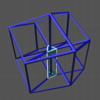This movie shows the orthographic view of a hypercube looking directly at a corner of the hypercube (i.e., along its long diagonal) while the hypercube is being sliced parallel to one of its edges. The initial slice is simply an edge, but since this edge is a member of three cubical faces of the hypercube, as the slice progresses it becomes a triangular prism. Its three rectangular sides are sweeping out the three cubes containing the initial edge. Each vertex of the hypercube is a member of four cubes, so at each endpoint of the initial edge there is one additional cube; the triangular faces come from these cubes as they are sliced corner first. As the slice continues, the prism grows until the top and bottom triangles are one third of the way through their respective cubes. At this point in the slicing sequence for a cube, the triangle's corners become cut off and the triangle becomes a hexagon. So the slice of the hypercube becomes a hexagonal prism. The original three rectangular sides of the prism have grown to their widest size as they slice through their respective cubes, and then start to shrink in width. At this point, the slice begins to enter three additional cubes (edge first) so three mores sides show up forming the hexagonal prism. These sides grow larger as the original ones grow smaller, and the prism again becomes triangular as the slice passes thorough three more of the vertices of the hypercube. Finally, the prism shrinks back to an edge opposite the original one.

` `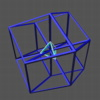This movie shows the orthographic view of a hypercube looking directly at a corner of the hypercube (i.e., along its long diagonal) while the hypercube is being sliced starting at a corner. The slice initially cuts off the corner at one end of the long diagonal, so the slice begins as a tetrahedron at the center of the figure. Each face of the tetrahedron is a slice of one of the corners of one of the four cubes that meet at this vertex. As the slice progresses, these faces grow and eventually pass through four of the vertices of the hypercube. At this point, the corners of the tetrahedron begin to be cut off as the slice starts to pass through the other four cubes in the hypercube. Meanwhile the original triangular faces are beginning to form hexagons as they slice farther through the original four cubes. At a point half-way through these cubes (or one-third of the way through the complete hypercube), these four cubes are sliced by regular hexagons, and the complete slice forms the Archimedean solid known as the truncated tetrahedron. It has four regular hexagonal faces and four equilateral triangular faces. Further along, the hexagonal faces become more triangular again, and the small triangular faces grow larger. Half-way through the slicing sequence for the hypercube, all eight faces are equilateral triangles forming a Platonic sold, the octahedron. At this point, all eight of the cubes in the hypercube are sliced in exactly the same way. As the slice moves further, the sequence repeats itself, but in reverse; it passes through the truncated tetrahedron, then the tetrahedron, which again shrinks to a vertex. This corner of the hypercube also appears to be at the center of the projection; since we are looking along the long diagonal, both the closest and farthest points on the hypercube are at the same location in the projection. Note that the final tetrahedron is inverted with respect to the one at the beginning of the slicing sequence.

` `

See the description of movie formats and plug-ins
if you are having difficulty getting these movies to play.

```
```